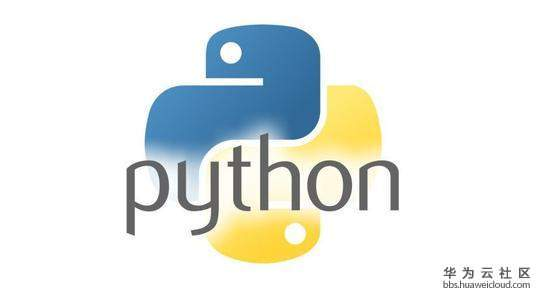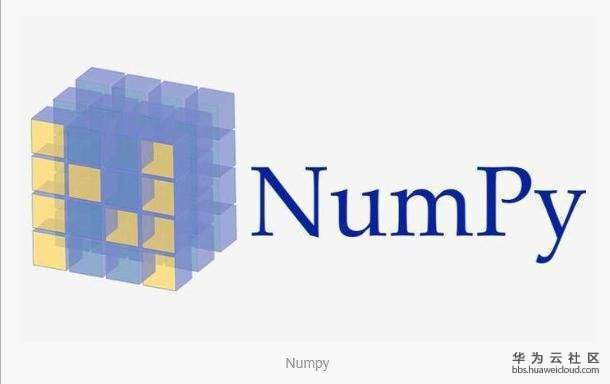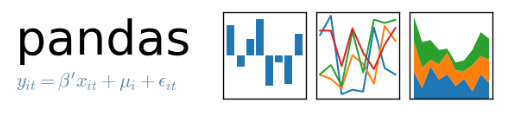[技术干货] 聚类分析算法——K-Means聚类算法

# 【Python算法】聚类分析算法——K-Means聚类算法

1. K-Means聚类算法过程

K-Means 是最常用的聚类方法之一，属于划分方法。
(1) 从N个样本数据中随机选取 K 个对象作为初始的聚类中心；
(2) 分别计算每个样本到各个聚类中心的距离，将对象分配到距离最近的聚类中；
(3) 所有对象分配完成后，重新计算 K 个聚类的中心；
(4) 与前一次计算得到的 K 个聚类中心比较，如果聚类中心发生变化，转过程（2），否则转过程（5）；
(5) 当质心不发生变化时停止并输出聚类结果。

2. K-Means聚类的主要特点

1.需要在开始时指定类别的数量，可以凭借直觉，也可以采用交叉验证或信息论的方法；
2.聚类的结果不是决定性的，即根据初始随机指定的几何中心不同，得到的最终聚类结果也会不同。

3. K-Means聚类语法结构

在 Python 中，Scikit-Learn 库提供了 KMeans 类，其构造函数为：

```sklearn.cluster.KMeans(
n_clusters=8,
init='k-means++',
n_init=10,
max_iter=300,
tol =0.0001,
precompute_distances='auto',
verbose=0,
random_state=None,
copy_x=True,
n_jobs=1,
algorithm='auto')```

其主要参数有：
(1) n_clusters：int 型，可选，默认为（8）。最终需要形成的几何中心数目，即类别个数；
(2) max_iter：int 型，默认为（300）。每次运行的最大迭代次数；
(3) n_init：int 型，默认为（10）。选用不同的初始化中心进行聚类的次数。

4.K-Means聚类主要属性有
(4) cluster_centers_：[n_clusters,n_features] 大小的数组。聚类中心的坐标；
(5) labels_：每个点的分类结果；
(6) inertia_：float 型，样本到最近聚合中心的距离和。

5.实验操作

5.1.操作系统
操作机：Linux_Ubuntu
操作机默认用户：root

5.2.实验工具
5.2.1.pythonPython是一种计算机程序设计语言。是一种动态的、面向对象的脚本语言，最初被设计用于编写自动化脚本(shell)，随着版本的不断更新和语言新功能的添加，越来越多被用于独立的、大型项目的开发。Python已经成为最受欢迎的程序设计语言之一。自从2004年以后，python的使用率呈线性增长。2011年1月，它被TIOBE编程语言排行榜评为2010年度语言。

由于Python语言的简洁性、易读性以及可扩展性，在国外用Python做科学计算的研究机构日益增多，一些知名大学已经采用Python来教授程序设计课程。例如卡耐基梅隆大学的编程基础、麻省理工学院的计算机科学及编程导论就使用Python语言讲授。

众多开源的科学计算软件包都提供了Python的调用接口，例如著名的计算机视觉库OpenCV、三维可视化库VTK、医学图像处理库ITK。而Python专用的科学计算扩展库就更多了，例如如下3个十分经典的科学计算扩展库：NumPy、SciPy和matplotlib，它们分别为Python提供了快速数组处理、数值运算以及绘图功能。因此Python语言及其众多的扩展库所构成的开发环境十分适合工程技术、科研人员处理实验数据、制作图表，甚至开发科学计算应用程序。

5.2.2.NumpyNumPy系统是Python的一种开源的数值计算扩展。这种工具可用来存储和处理大型矩阵，比Python自身的嵌套列表（nested list structure)结构要高效的多（该结构也可以用来表示矩阵（matrix））。
NumPy（Numeric Python）提供了许多高级的数值编程工具，如：矩阵数据类型、矢量处理，以及精密的运算库。专为进行严格的数字处理而产生。多为很多大型金融公司使用，以及核心的科学计算组织如：Lawrence Livermore，NASA用其处理一些本来使用C++，Fortran或Matlab等所做的任务。

5.2.3.scikit-learnscikit-learn，Python 中的机器学习，简单高效的数据挖掘和数据分析工具，可供大家使用，可在各种环境中重复使用，建立在 NumPy，SciPy 和 matplotlib 上开放源码，可商业使用 - BSD license。

5.2.4.MatplotlibMatplotlib 是一个 Python 的 2D绘**，它以各种硬拷贝格式和跨平台的交互式环境生成出版质量级别的图形。通过 Matplotlib，开发者可以仅需要几行代码，便可以生成绘图，直方图，功率谱，条形图，错误图，散点图等。

5.2.5.pandasPython Data Analysis Library 或 pandas 是基于NumPy 的一种工具，该工具是为了解决数据分析任务而创建的。Pandas 纳入了大量库和一些标准的数据模型，提供了高效地操作大型数据集所需的工具。pandas提供了大量能使我们快速便捷地处理数据的函数和方法。你很快就会发现，它是使Python成为强大而高效的数据分析环境的重要因素之一。

5.3导入所需要的库:numpy,matplotlib.pyplot,pandas

```# Importing the libraries
import numpy as np
import matplotlib.pyplot as plt
import pandas as pd```

5.4.加载数据集

```# Importing the dataset
dataset = pd.read_csv('数据集路径.CSV')
X = dataset.iloc[:, 3:5].values```5.5.使用sklearn中的k-means方法对数据进行聚类，并对其参数选择进行评估

```# Using the elbow method to find the optimal number of clusters
from sklearn.cluster import KMeans
wcss = []
for i in range(1,11):
kmeans = KMeans(n_clusters = i, max_iter = 300, n_init = 10, init = 'k-means++', random_state = 0)
kmeans.fit(X)
wcss.append(kmeans.inertia_)
plt.plot(range(1,11), wcss)
plt.title('The Elbow Method')
plt.xlabel('Number of Clusters')
plt.ylabel('WCSS')
plt.show()```

WCSS下降曲线如下：在选择参数时，选择速度转换最快的阶段与下一阶段的转折点。
由图可知：聚类数量为5。

5.6.将k-means算法用于数据集

```# Applying the k-means to the mall dataset
kmeans = KMeans(n_clusters = 5, max_iter = 300, n_init = 10, init = 'k-means++', random_state = 0)
y_kmeans = kmeans.fit_predict(X)```

5.7.可视化聚类结果

```# Visualizing the clusters
plt.scatter(X[y_kmeans == 0, 0], X[y_kmeans == 0, 1], s = 100, c = 'red', label = 'Careful')
plt.scatter(X[y_kmeans == 1, 0], X[y_kmeans == 1, 1], s = 100, c = 'blue', label = 'Standard')
plt.scatter(X[y_kmeans == 2, 0], X[y_kmeans == 2, 1], s = 100, c = 'green', label = 'Target')
plt.scatter(X[y_kmeans == 3, 0], X[y_kmeans == 3, 1], s = 100, c = 'cyan', label = 'Careless')
plt.scatter(X[y_kmeans == 4, 0], X[y_kmeans == 4, 1], s = 100, c = 'magenta', label = 'Sensible')
plt.scatter(kmeans.cluster_centers_[:, 0],  kmeans.cluster_centers_[:, 1], s = 300, c = 'yellow', label = 'Centroids')
plt.title('Clusters of clients')
plt.xlabel('Annual Income (k\$)')
plt.ylabel('Spending Score (1-100)')
plt.legend()
plt.show()```